A Maple-graded question uses the MapleTM language to evaluate student responses.

• Accepting a wider range of mathematical expressions as responses
• Questions with more than one answer or an infinite number of answers
• Questions requiring a powerful response-equivalence checker

This question type uses the computational power and plotting capabilities of the Maple mathematics engine to work with algebra, calculus, differential equations, discrete mathematics, numerical computation, and many other areas of mathematics.

As well, you can use functions and routines that are contained in a separate Maple repository (Maple library).

You can choose from two Maple-graded question sub-types when authoring a Maple-graded question.

You'll define the sub-type that you're working with by selecting an expression type during question authoring:

• The formula sub-type is authored by selecting the formula expression type
• The Maple syntax sub-type is authored by selecting the Maple syntax expression type

TIP: Check out Choose a Maple-graded question sub-type for a comparison of the two available Maple-graded question sub-types.

TIP: All questions are authored using the Question Editor. Check out Author a question to learn how to access the Question Editor.

## To author a Maple-graded question

To author a Maple-graded question from the Question Editor:

1. Enter the question statement in the Question Text pane of the Question Editor.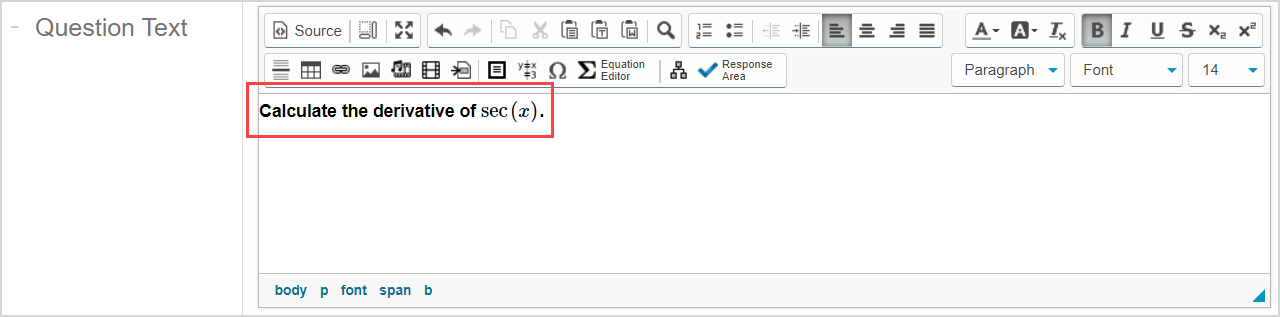TIP: Check out Use the Visual Editor for details on how to use this toolbar to the fullest to control the visual appearance of your Maple-graded question text.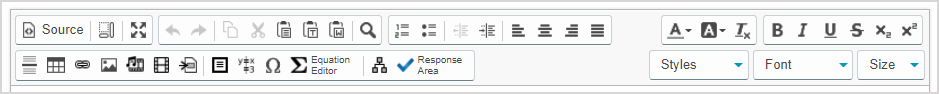1. Click Response Areato select the type of response area for this part of the question.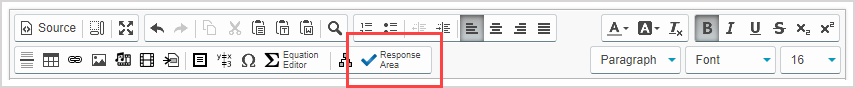NOTE: A question can have multiple question parts. Each question part have a different type of response area. Example — A question could have the first response area as a Maple-graded response area, and a second response area as multiple choice.

1. Click Maple-graded from the list of response area types.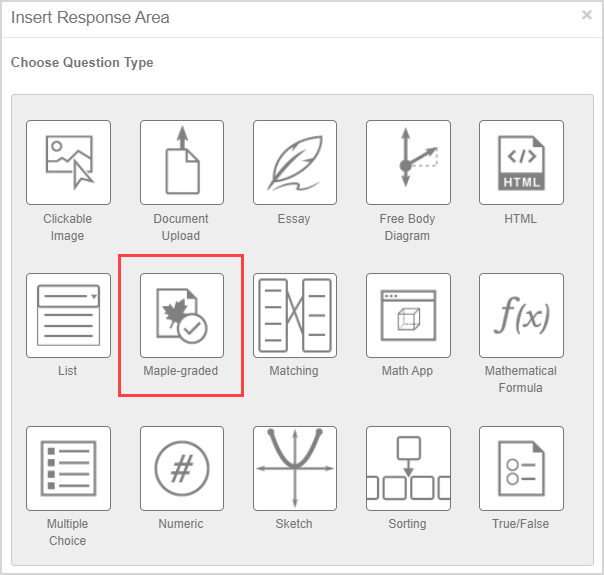1. Assign a weight to the response area. The default response area weighting is 1.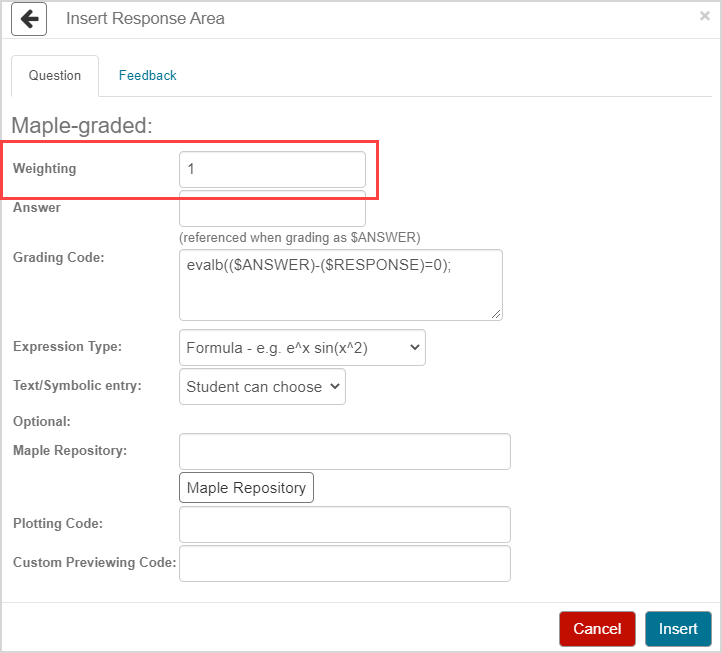TIP: The response area weight can be any integer greater than 0. Response area weighting is proportionate to the question total. Example — A question with two response areas could have the first response area weighted as 2, and the second response area weighted as 4. This means that the second response area will be worth twice as much as the first response area when calculating the total grade for the question.

1. Enter the correct answer in Maple syntax (regardless of the Maple-graded sub-type you plan on authoring) that you want to be displayed to your students (Example — when a student is viewing their graded attempt) in the Answer field.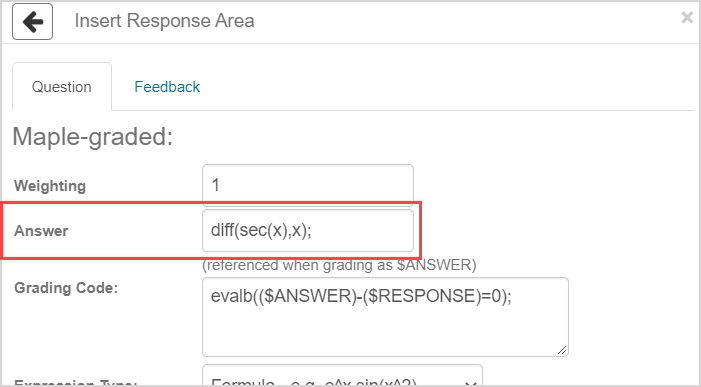TIP: Follow these rules while defining the correct response in the Answer field:

• The last line of code must evaluate to an expression which will be stored to the variable \$ANSWER.
• Complete each line of code in the Answer field with a semicolon (;).
• Add parentheses when appropriate (Example(x + y) / 2).
• Use the long form name for all package functions (ExampleVectorCalculus[ArcLength]).

TIP: (A) Simply entering the correct answer into the Answer field will display the correct answer but it won't be displayed to students with properly formatted symbolic math. (B) You can use the Maple command printf(MathML[ExportPresentation](a)); in the Answer field or the Algorithm pane, where a is the correct answer in Maple, to present the correct answer to your students in properly formatted symbolic math.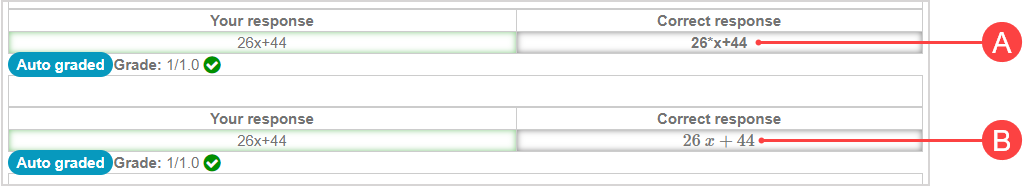However, if you decide to use the printf(MathML[ExportPresentation](a)); command, then \$ANSWER can't be used in your grading code. Check out the Example: Formatted correct answer section of the Author a Maple syntax sub-type help topic to see how to use this formatting code.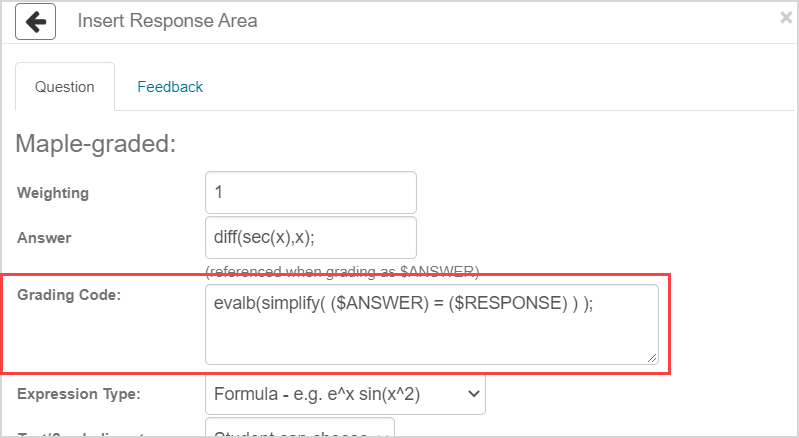TIP: Follow these rules while defining the correct response in the Grading Code field:

• Use valid Maple code to evaluate the answer.
• Use \$RESPONSE to represent the value of the student’s response in your code.
• Before your code is processed by Maple, \$RESPONSE is replaced by the student response.
• The last line of your grading code must evaluate to a Boolean value (true or false) or a floating-point number between 0.0 and 1.0 for partial grading.
• By default, use the Maple command evalb((\$ANSWER)-(\$RESPONSE)=0); as indicated, where \$RESPONSE represents the student's response and \$ANSWER represents the correct answer.
• If you choose to alter the default grading syntax, complete each line of code with a semicolon (;).

• Use the long form name for all package functions (ExampleVectorCalculus[ArcLength]).

TIP: It's recommended that you test your Maple grading code within a Maple worksheet to ensure that it behaves as expected.

1. Define the question sub-type using the Expression Type drop-down list. The sub-type determines the format that the student can use to enter their response. Choose from:

• Formula — The student can choose between text or symbolic entry but can't use Maple syntax.
• Maple Syntax — The student must use the entry mode that you define (and must use Maple syntax if text entry mode is required).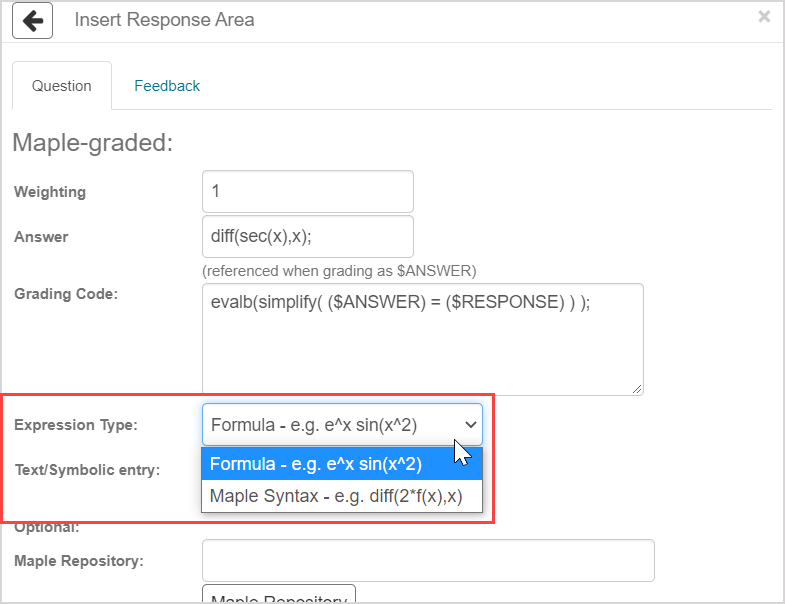TIP: Check out Choose a Maple-graded question sub-type for a comparison of the two available Maple-graded question sub-types.

1. Select the student entry mode from the Text/Symbolic entry drop-down list.

NOTE: If text entry mode is being used, the student will type their response into a text field. If symbol entry mode is being used, the student will enter their response using the Equation Editor's symbol palettes.

• Did you select Formula as the expression at step 7? Then the Student can choose entry mode is selected by default and can't be changed.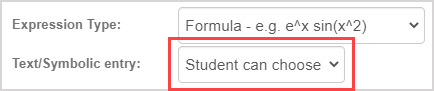• Did you select Maple Syntax as the expression type at step 7? Then you'll define if the question requires the student to use Text entry only or Symbol entry only.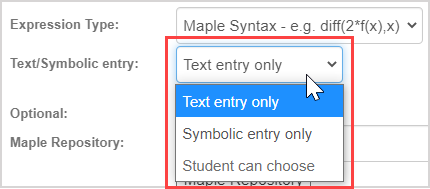IMPORTANT: Your students won't be able to tell if a question is authored as a Maple-graded Maple syntax question. You'll need to provide explicit instructions in your question statement if Maple syntax is required for their response.

1. (Optional) Click Maple Repository to upload a Maple library from your personal Maple library archives into your question. The file path to your library will autofill after selecting it from your repository.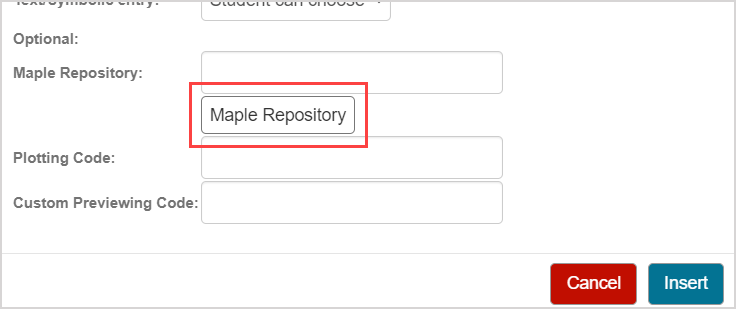NOTE: The Maple repository is used to access your Maple library files (.mla file extension). Maple library files are uploaded to your question using the Class File Manager(check out Manage folders in the Class File Manager). The Maple libname variable will automatically be set to access the archive files specified when executing the Maple code associated with the question. When setting up multiple archives inside the same directory, ensure that there are no name conflicts. If you want to share your question, you must also share the Maple files.

NOTE: Maple libraries aren't available for use in algorithm variable definition statements.

1. (Optional) Enter a plotting code to enable automatic plotting of the student response.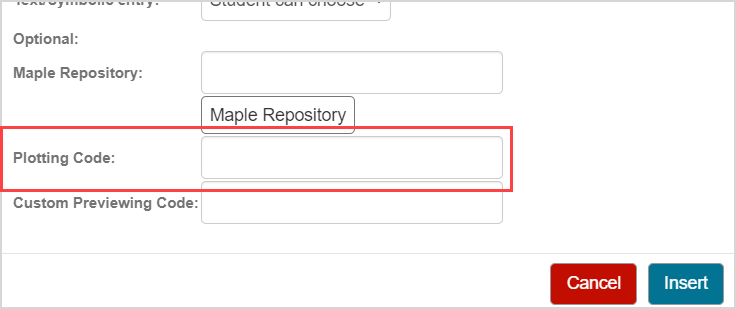TIP: Before your code is processed by Maple, \$RESPONSE is replaced by the student's response. When defining your plotting code:

• Use \$RESPONSE to represent the student's response in your code
• The last line must be a Maple plot command

TIP: Check out the Example: Plotting code and infinite number of correct responses section of the Author a formula (Maple-graded) sub-type help topic to see how to use plotting code.

NOTE: When plotting code is defined, the student will be able to click the Plot icon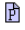associated with the response area for a preview of their plotted response.

1. (Optional) If the student is using the text entry mode for their response, you can enter custom previewing code to change how the student's response is displayed when they click the Preview icon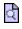to preview their response (Example — a custom previewing code could be defined to prevent simplification of the student's response).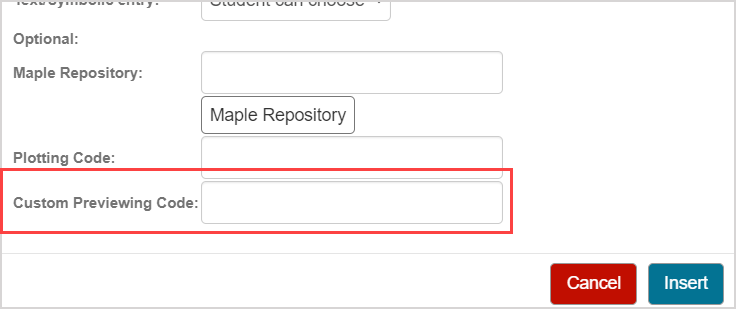NOTE: Before your code is processed by Maple, \$RESPONSE is replaced by the student's response.

TIP: Check out the Example: Custom previewing code section in the Author a formula (Maple-graded) sub-type to see how to use this custom previewing code.

1. Click Insert to add this response area to the question.1. The response area is inserted into your question.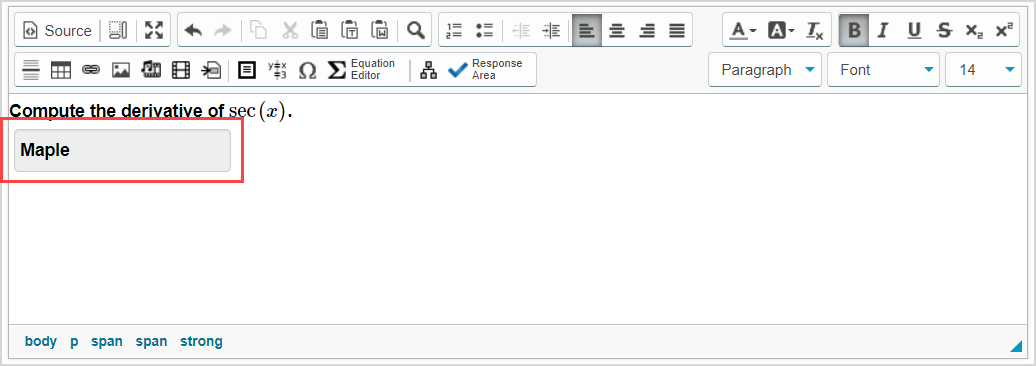1. Click Preview to trial your new question to ensure that it behaves as expected.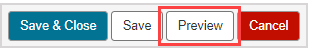TIP: You first have to click the save iconif you're creating this question from within an Activity Editor. After the question has been saved, you can then click the preview icon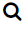to ensure that your new question behaves as expected.

1. Click Save to save your new question. Your new question is now stored in the Questions pane of the Content Repository.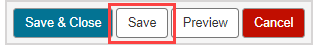NOTE: The button panel will appear differently if you are authoring your question from within an Activity Editor, but the Save option is still available.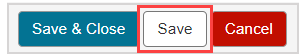NOTE: evalb( (\$ANSWER) – (\$RESPONSE) = 0 ); is the default grading command.

Both Maple-graded sub-types allow you to use Maple functions and expressions in the grading code (Example — trigonometric functions, log10, ln, abs, sqrt, diff, int, LinearAlgebra[Determinant], etc.).

In addition to the full range of Maple functions, you can use programming constructs to evaluate responses.

IMPORTANT: You may need to write Maple code for responses that Möbius interprets as formulas when working with the formula sub-type of a Maple-graded question. Be sure to structure your grading code to be specific for accepting a numerical response or evaluating a function. Example — If the question is What is the value of sin(Pi/4)?, you must use code to ensure that the student doesn't simply enter sin(Pi/4) (which Möbius accepts and passes to the Maple engine). The correct response is sqrt(2)/2 which is Möbius formula and a Maple command.